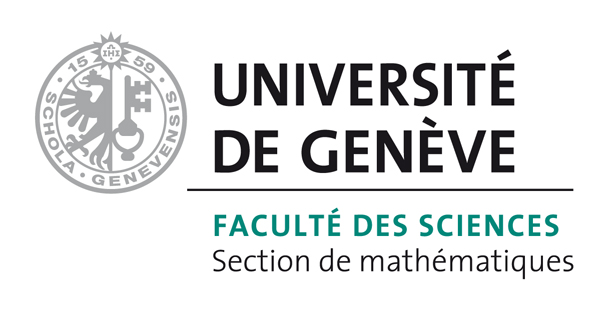# Bart Vandereycken## Embedded geometry of the set of symmetric positive semidefinite matrices of fixed rank

by , ,

#### Abstract:

This paper deals with the Riemannian geometry of the set of symmetric positive semidefinite matrices of fixed rank. This set is studied as an embedded submanifold of the real matrices equipped with the usual Euclidean metric. With this structure, we derive expressions of the tangent space and geodesics of the manifold, suitable for efficient numerical computations.

#### Reference:

B. Vandereycken, P.-A. Absil, S. Vandewalle, "Embedded geometry of the set of symmetric positive semidefinite matrices of fixed rank", In SSP09, pp. 389-392, 2009.

#### Bibtex Entry:

@inproceedings{Vandereycken_AV_2009,
Abstract = {This paper deals with the Riemannian geometry of the set of symmetric positive semidefinite matrices of fixed rank. This set is studied as an embedded submanifold of the real matrices equipped with the usual Euclidean metric. With this structure, we derive expressions of the tangent space and geodesics of the manifold, suitable for efficient numerical computations.},
Author = {Vandereycken, B. and Absil, P.-A. and Vandewalle, S.},
Booktitle = {SSP09},
Doi = {10.1109/SSP.2009.5278558},
Pages = {389--392},
Pdf = {http://www.unige.ch/math/vandereycken/papers/preprint_Vandereycken_AV_2009.pdf},
Title = {Embedded geometry of the set of symmetric positive semidefinite matrices of fixed rank},
Year = {2009}}